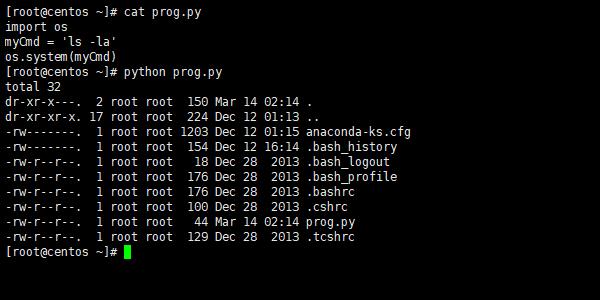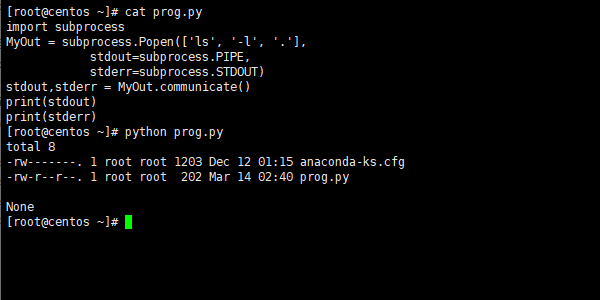Python是一款非常出色的脚本语言，越来越多的系统管理员开始使用Python将日常工作自动化。

```import os
myCmd = 'ls -la'
os.system(myCmd)``````import os
myCmd = 'ls -la > out.txt'
os.system(myCmd)```

```import os
print(myCmd)```

```import subprocess
subprocess.call("ls")```

```import subprocess
subprocess.call(["ls", "-l", "."])```

```import subprocess
MyOut = subprocess.Popen(['ls', '-l', '.'],
stdout=subprocess.PIPE,
stderr=subprocess.STDOUT)
stdout,stderr = MyOut.communicate()
print(stdout)
print(stderr)```